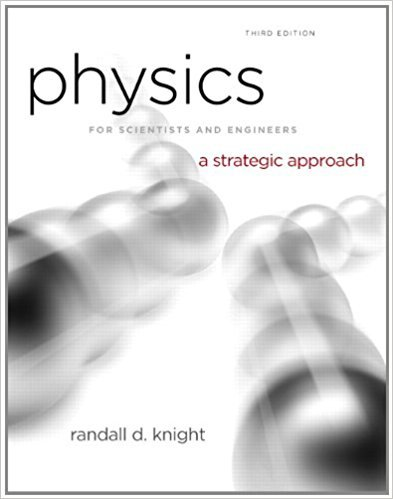×
Get Full Access to Physics For Scientists And Engineers: A Strategic Approach With Modern Physics - 3 Edition - Chapter 5 - Problem 31p
Get Full Access to Physics For Scientists And Engineers: A Strategic Approach With Modern Physics - 3 Edition - Chapter 5 - Problem 31p

×

# A single force with x -component Fx acts on a 500 g objectISBN: 9780321740908 69

## Solution for problem 31P Chapter 5

Physics for Scientists and Engineers: A Strategic Approach with Modern Physics | 3rd Edition

• Textbook Solutions
• 2901 Step-by-step solutions solved by professors and subject experts
• Get 24/7 help from StudySoup virtual teaching assistantsPhysics for Scientists and Engineers: A Strategic Approach with Modern Physics | 3rd Edition

4 5 1 370 Reviews
13
0
Problem 31P

Problem 31P

A single force with x -component Fx acts on a 500 g object as it moves along the x -axis. The object’s acceleration graph (ax versus t ) is shown in FIGURE. Draw a graph of Fx versus t.

FIGUREStep-by-Step Solution:

Solution 31P

Step 1 of 3

The objective here is to plot a Forceversus time t of an object of constant known mass from its acceleration-time graph.

Step 2 of 3

Step 3 of 3

##### ISBN: 9780321740908

Physics for Scientists and Engineers: A Strategic Approach with Modern Physics was written by and is associated to the ISBN: 9780321740908. Since the solution to 31P from 5 chapter was answered, more than 2244 students have viewed the full step-by-step answer. The full step-by-step solution to problem: 31P from chapter: 5 was answered by , our top Physics solution expert on 08/30/17, 04:34AM. This full solution covers the following key subjects: versus, Object, graph, figure, Force. This expansive textbook survival guide covers 17 chapters, and 1439 solutions. This textbook survival guide was created for the textbook: Physics for Scientists and Engineers: A Strategic Approach with Modern Physics, edition: 3. The answer to “A single force with x -component Fx acts on a 500 g object as it moves along the x -axis. The object’s acceleration graph (ax versus t ) is shown in FIGURE. Draw a graph of Fx versus t.FIGURE” is broken down into a number of easy to follow steps, and 39 words.

Unlock Textbook Solution